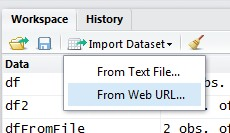The Read.Table function reads a file in table format and creates a data frame from it, with cases corresponding to lines and variables to fields in the file.

## Syntax

``````read.table(
file,
sep = "",
quote = "\"'",
dec = ".",
row.names,
col.names,
as.is = !stringsAsFactors,
na.strings = "NA",
colClasses = NA,
nrows = -1,
skip = 0,
check.names = TRUE,
fill = !blank.lines.skip,
strip.white = FALSE,
blank.lines.skip = TRUE,
comment.char = "#",
allowEscapes = FALSE,
flush = FALSE,
stringsAsFactors = default.stringsAsFactors(),
fileEncoding = "",
encoding = "unknown",
text
)```
```

where:

• file can be a file, an Url or a connection.
• sep is a string indicating how the columns are separated
• colClasses, a character vector indicating the class of each column in the dataset
• nrows, the number of rows in the dataset
• comment.char, a character string indicating the comment character
• skip, the number of lines to skip from the beginning
• stringsAsFactors, should character variables be coded as factors?

## Performance

• figure out: colclasses (what type of variable is in each column of the table)
• check if each line is a comment: comment.char (comment.char = “” disable it)

By giving R all these parameters will make R run faster as it don't need to perform them.

## Memory

The dataset must no be larger than the amount of your RAM.

1,000,000 rows, 10 columns with numeric data = 1,000,000 * 10 * 8 bytes = 76 Mb

## Options

### colClasses

````colClasses = "numeric"`
```

To figure out the classes of each column, you can use this snippets:

``````mySubsetDataTable = read.table("myFile.txt", nrows = 100)
classes = sapply(mySubsetDataTable, class)
myDataTable = read.table("myFile.txt", colClasses = classes)```
```

### nrows

See the Linux tool wc on how to calculate the number of lines in a file.

Setting nrows will help with memory usage.

Recommended PagesR - Csv

csv read.csv is identical to read.table except that the default separator is a comma is the same as - Read Rectangular Data (Tabular)R - Data frame Object

A data frame is a logical implementation of a table in a relational database A data frame inherits all the property and function of an object. It has a list of variables of the same number of rows with...R - Excel (xlsx )